Some Important Definitions

(1) Time period : It is the least interval of time after which the periodic motion of a body repeats itself.

S.I. units of time period is second.

(2) Frequency : It is defined as the number of periodic motions executed by body per second. S.I unit of frequency is hertz (Hz).

(3) Angular Frequency : Angular frequency of a body executing periodic motion is equal to product of frequency of the body with factor 2p. Angular frequency w = 2 p n

S.I. units of w is Hz [S.I.] w also represents angular velocity. In that case unit will be rad/sec.

(4) Displacement : In general, the name displacement is given to a physical quantity which undergoes a change with time in a periodic motion.

Examples :

(i) In an oscillation of a loaded spring, displacement variable is its deviation from the mean position.

(ii) During the propagation of sound wave in air, the displacement variable is the local change in pressure

(iii) During the propagation of electromagnetic waves, the displacement variables are electric and magnetic fields, which vary periodically.

(5) Phase : phase of a vibrating particle at any instant is a physical quantity, which completely express the position and direction of motion, of the particle at that instant with respect to its mean position.

In oscillatory motion the phase of a vibrating particle is the argument of sine or cosine function involved to represent the generalised equation of motion of the vibrating particle.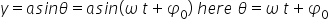phase of vibrating particle.

(i) Initial phase or epoch : It is the phase of a vibrating particle at t = 0. in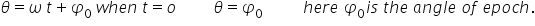(ii) Same phase : Two vibrating particle are said to be in same phase, if the phase difference between them is an even multiple of p or path difference is an even multiple of (l / 2) or time interval is an even multiple of (T / 2) because 1 time period is equivalent to 2p rad or 1 wave length (l)

(iii) Opposite phase : When the two vibrating particles cross their respective mean positions at the same time moving in opposite directions, then the phase difference between the two vibrating particles is 180o

Opposite phase means the phase difference between the particle is an odd multiple of p (say p, 3p, 5p, 7p…..) or the path difference is an odd multiple of l (say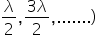or the time interval is an odd multiple of (T / 2).

(iv) Phase difference :  If two particles performs S.H.M and their equation are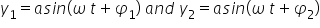then phase difference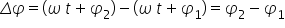Related Keywords